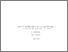# Bayesian Inverse Regression and Discrimination: An Application of Credibility Theory

Avenhaus, R. & Jewell, W.S. (1975). Bayesian Inverse Regression and Discrimination: An Application of Credibility Theory. IIASA Research Memorandum. IIASA, Laxenburg, Austria: RM-75-027Preview Text RM-75-027.pdf Download (654kB) | Preview

## Abstract

Many measurement problems can be formulated as follows: a certain linear relationship between two variables is to be estimated by using pairs of input and output data; the value of an unknown input variable is then estimated, given an observation of the corresponding output variable. This problem is often referred to as inverse regression or discrimination.

In this paper, we formulate a general Bayesian calibration and measurement model for this problem, in which prior information is assumed to be available on the relationship parameters, the possible values of the unknown input, and the output observation error. Simplified and easily interpreted formulae for estimating the posterior mean and variance of the input are then developed using the methods of credibility theory, a linearized Bayesian analysis developed originally for insurance estimation problems. A numerical example of the calibration of a calorimeter to measure nuclear material is given.

Item Type: Monograph (IIASA Research Memorandum) System and Decision Sciences - Core (SDS) IIASA Import 15 Jan 2016 01:43 27 Aug 2021 17:08 https://pure.iiasa.ac.at/489

### Actions (login required)View Item